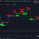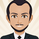1372 views
1372
Adaptive Moving Average indicator script. This indicator was originally developed by Vitali Apirine (Stocks & Commodities V.36:5: Adaptive Moving Averages).
Nov 05
Release Notes: Refactored
Dec 27
Release Notes:
A list of Free indicators: https://bit.ly/2S7EPuN
A list of Paid indicators: https://bit.ly/2SQOhDF

Tip Jar
PayPal: https://www.paypal.me/alexeverget
ETH: 0x2393f8a932828ef0274ebbc4f873152837d3ab5b"

inputs:
Price( numericseries ),
EffRatioLength( numericsimple ),
FastAvgLength( numericsimple ), { this input assumed to be a constant >= 1 }
SlowAvgLength( numericsimple ) ; { this input assumed to be a constant >= 1 }

{ Eff = Efficiency }

variables:
NetChg( 0 ),
TotChg( 0 ),
EffRatio( 0 ),
ScaledSFSqr( 0 ),
SlowAvgSF( 2 / ( SlowAvgLength + 1 ) ),
FastAvgSF( 2 / ( FastAvgLength + 1 ) ),
SFDiff( FastAvgSF - SlowAvgSF ) ,
pds(0),
MLTP(0),
SSC(0);

pds = EffRatioLength + 1;
MLTP = AbsValue(Price - Lowest(low, pds)) - (highest(high, pds) - price) / (highest(high, pds) - lowest(low, pds));
SSC = MLTP * (FastAvgSF - SlowAvgSF) + SlowAvgSF;

"

This is what I have so far, when plugged into Tradestation along with an indicator to plot it the AMA comes out significantly different than the one on my chart from tradingview. Thanks in advance.Hi,
Function: _AMA

// TASC APR 2018
// _AMA
// Function
// Vitali Apirine

inputs:
Periods( numericsimple ),
FastAvgLength( numericsimple ),
SlowAvgLength( numericsimple ) ;

variables:
PDS( Periods + 1 ),
FastSC( 2 / ( FastAvgLength + 1 ) ),
SlowSC( 2 / ( SlowAvgLength + 1 ) ),
SSC( 0 ),
CST( 0 ),
MLTP( 0 );

MLTP = AbsValue( ( Close - Lowest( Low, PDS ) )
- ( Highest( High, PDS ) - Close ))
/ ( Highest( High, PDS ) - Lowest( Low, PDS ) ) ;

SSC = MLTP * ( FastSC - SlowSC ) + SlowSC ;

CST = Square( SSC ) ;

if CurrentBar = 1 then
_AMA = Close + CST * ( Close - Close )
else
_AMA = _AMA + CST * ( Close - _AMA ) ;

--------------------------------------------------------------------

// TASC APR 2018
// Indicator
// Vitali Apirine

inputs:
Periods( 10 ),
FastAvgLength( 2 ),
SlowAvgLength( 30 ) ;

variables:
AMA( 0 ),
KAMA( 0 ) ;

AMA = _AMA( Periods, FastAvgLength,
SlowAvgLength ) ;
FastAvgLength, SlowAvgLength ) ;

Plot1( AMA, "AMA", Cyan ) ;
Plot2( KAMA, "KAMA", Magenta ) ;